Question

When a 25.9 mL sample of a 0.317 M aqueous nitrous acid solution is titrated with a 0.458 M aqueous sodium hydroxide solution, what is the pH after 26.9 mL of sodium hydroxide have been added? Please show work!

millimoles of HNO2 = 25.9 x 0.317 = 8.21

millimoles of NaOH = 0.458 x 26.9 = 12.32

Ka of HNO2 = 5.6×10–4

pKa of HNO2 = 3.25

HNO2 + NaOH   -----------> NaNO2 + H2O

8.21      12.32                            0             0

0          4.11                            8.21

here strong acid remains. so

[NaOH] = 4.11 / (25.9 + 26.9) = 0.0778

pOH = -log [OH-] = -log (0.0778)

poH = 1.11

pH = 12.89

#### Earn Coins

Coins can be redeemed for fabulous gifts.

Similar Homework Help Questions
• ### When a 26.7 ml sample of a 0.361 M aqueous nitrous acid solution is titrated with...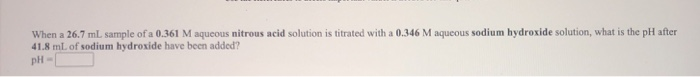When a 26.7 ml sample of a 0.361 M aqueous nitrous acid solution is titrated with a 0.346 M aqueous sodium hydroxide solution, what is the pH after 41.8 mL of sodium hydroxide have been added? pH- A 47.8 ml sample of a 0.422 M aqueous nitrous acid solution is titrated with a 0.473 M aqueous sodium hydroxide solution. What is the pH after 17.2 mL of base have been added? pH-

• ### When a 28.9 mL sample of a 0.453 M aqueous nitrous acid solution is titrated with...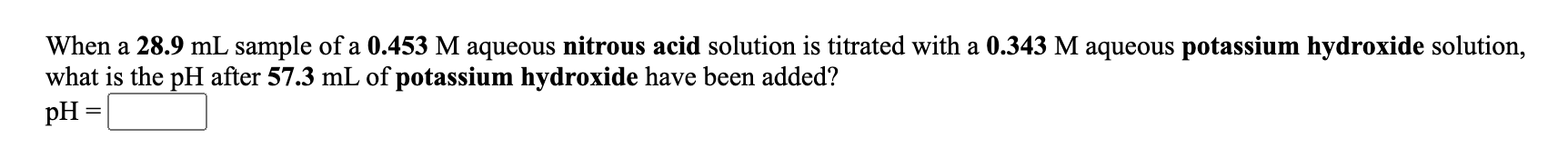When a 28.9 mL sample of a 0.453 M aqueous nitrous acid solution is titrated with a 0.343 M aqueous potassium hydroxide solution, what is the pH after 57.3 mL of potassium hydroxide have been added? When a 28.9 mL sample of a 0.453 M aqueous nitrous acid solution is titrated with a 0.343 M aqueous potassium hydroxide solution, what is the pH after 57.3 mL of potassium hydroxide have been added? pH =

• ### (1) A 31.4 mL sample of a 0.531 M aqueous nitrous acid solution is titrated with...

(1) A 31.4 mL sample of a 0.531 M aqueous nitrous acid solution is titrated with a 0.305 M aqueous barium hydroxide solution. What is the pH after 10.4 mL of base have been added? (2) When a 28.7 mL sample of a 0.424 M aqueous hydrofluoric acid solution is titrated with a 0.352 M aqueous sodium hydroxide solution, what is the pH after 51.9 mL of sodium hydroxide have been added?

• ### 28) When a 21.7 mL sample of a 0.378 M aqueous nitrous acid solution is titrated...

28) When a 21.7 mL sample of a 0.378 M aqueous nitrous acid solution is titrated with a 0.496 M aqueous sodium hydroxide solution, what is the pH after 24.8 mL of sodium hydroxide have been added? pH = ___

• ### 1.When a 15.1 mL sample of a 0.479 M aqueous nitrous acid solution is titrated with...

1.When a 15.1 mL sample of a 0.479 M aqueous nitrous acid solution is titrated with a 0.483 M aqueous sodium hydroxide solution, what is the pH after 22.5 mL of sodium hydroxide have been added? 2.A 21.6 mL sample of 0.214 M diethylamine, (C2H5)2NH, is titrated with 0.256 M perchloric acid. The pH before the addition of any perchloric acid is

• ### a. When a 20.0 mL sample of a 0.431 M aqueous nitrous acid solution is titrated...

a. When a 20.0 mL sample of a 0.431 M aqueous nitrous acid solution is titrated with a 0.369 M aqueous sodium hydroxide solution, what is the pH at the midpoint in the titration? b. A 26.4 mL sample of a 0.351 M aqueous nitrous acid solution is titrated with a 0.345 M aqueous potassium hydroxide solution. What is the pH at the start of the titration, before any potassium hydroxide has been added?

• ### A 46.2 mL sample of a 0.345 M aqueous nitrous acid solution is titrated with a...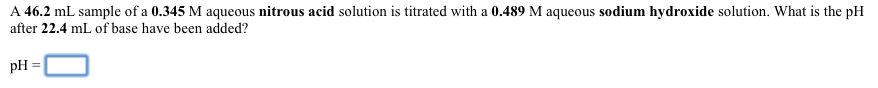A 46.2 mL sample of a 0.345 M aqueous nitrous acid solution is titrated with a 0.489 M aqueous sodium hydroxide solution. What is the pH after 22.4 mL of base have been added? pH =

• ### When a 23.3 mL sample of a 0.443 M aqueous nitrous acid solution is titrated with...

When a 23.3 mL sample of a 0.443 M aqueous nitrous acid solution is titrated with a 0.305 M aqueous barium hydroxide solution, what is the pH after 25.4 mL of barium hydroxide have been added? pH =

• ### When a 23.8 mL sample of a 0.443 M aqueous hydrofluorie acid solution is titrated with...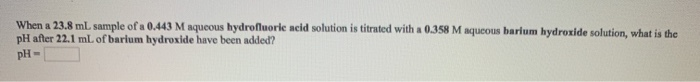When a 23.8 mL sample of a 0.443 M aqueous hydrofluorie acid solution is titrated with a 0.358 M aqueous barium hydroxide solution, what is the pH after 22.1 mL of barium hydroxide have been added? pH- What is the pH at the equivalence point in the titration of a 19.7 mL sample of a 0.376 M aqueous nitrous acid solution with a 0.447 M aqueous sodium hydroxide solution? pH- When a 20.1 mL sample of a 0.417 M aqueous...

• ### When a 23.0 mL sample of a 0.311 M aqueous nitrous acid solution is titrated with...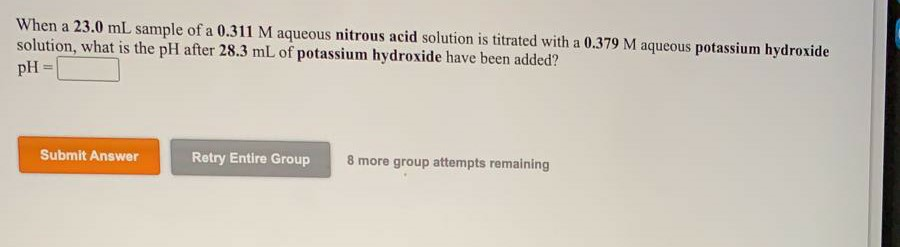When a 23.0 mL sample of a 0.311 M aqueous nitrous acid solution is titrated with a 0.379 M aqueous potassium hydroxide solution, what is the pH after 28.3 mL of potassium hydroxide have been added? pH = Submit Answer Retry Entire Group 8 more group attempts remaining